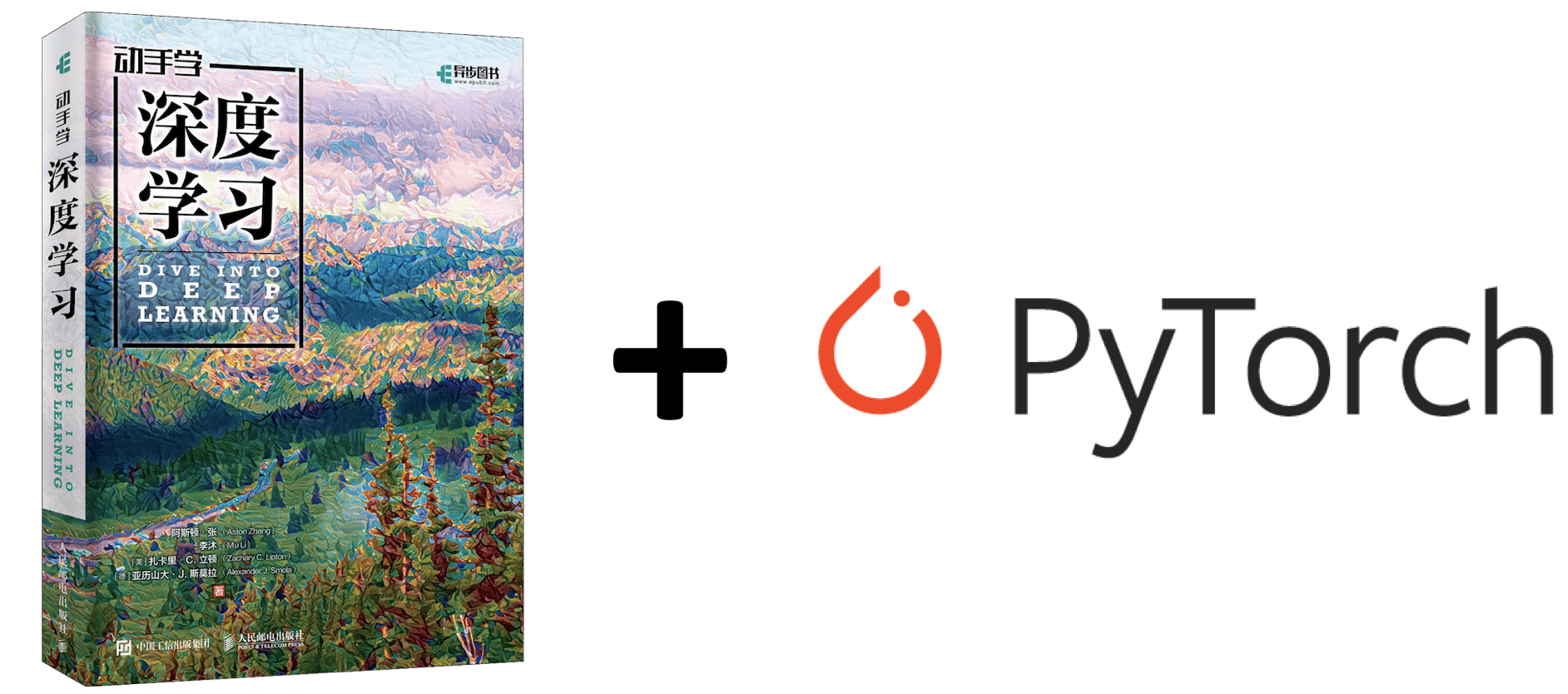Part C 包含：

• § 7 优化算法
• 优化与深度学习，优化存在的挑战
• 梯度下降（略）
• § 8 计算性能
• 多 GPU 计算
• 多 GPU 计算时模型的保存与加载
• § 10 NLP
• Word2Vec, fastText, GloVe
• Encoder-Decoder, Beam Search
• Attention

## 优化算法

### 优化与深度学习

• 局部最小值

（之前的笔记中记录已十分详细，此处略去）

### 动量法

指数移动加权平均法，是指各数值的加权系数随时间呈指数式递减，越靠近当前时刻的数值加权系数就越大

指数移动加权平均较传统的平均法来说，一是不需要保存过去所有的数值；二是计算量显著减小。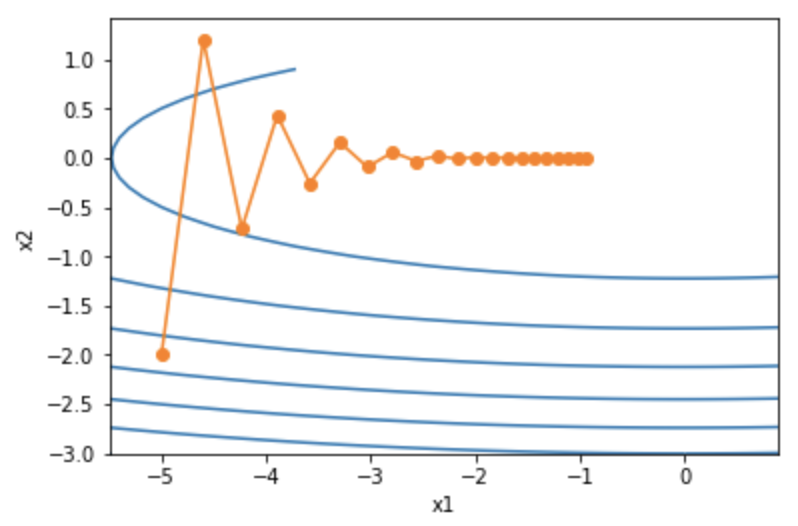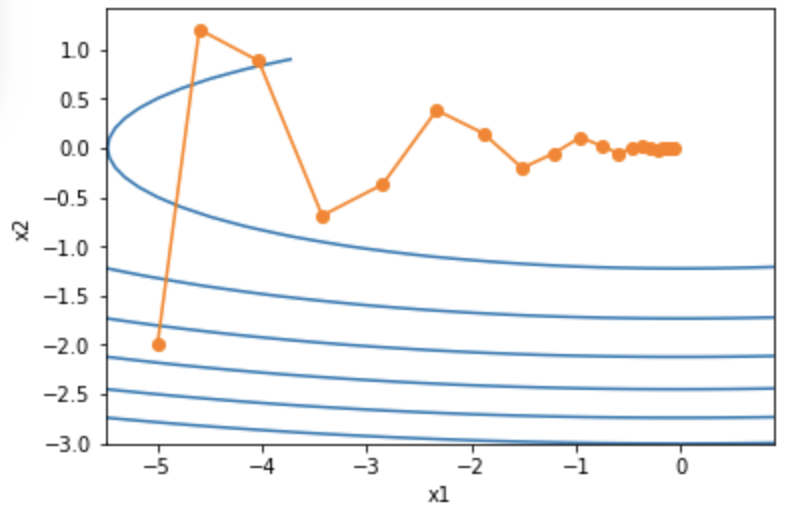d2l.train_pytorch_ch7(torch.optim.SGD, {'lr': 0.004, 'momentum': 0.9},
features, labels)

AdaGrad 算法会使用一个小批量随机梯度 $\boldsymbol{g}_t$​​ 按元素平方的累加变量 $\boldsymbol{s}_t$​​。在时间步 0，AdaGrad 将 $\boldsymbol{s}_0$​​ 中每个元素初始化为 0。在时间步 $t$​​，首先将小批量随机梯度 $\boldsymbol{g}_t$​​ 按元素平方后累加到变量 $\boldsymbol{s}_t$​​：

d2l.train_pytorch_ch7(torch.optim.Adagrad, {'lr': 0.1}, features, labels)

### RMSProp

d2l.train_pytorch_ch7(torch.optim.RMSprop, {'lr': 0.01, 'alpha': 0.9},
features, labels)

AdaDelta 算法也像 RMSProp 算法一样，使用了小批量随机梯度 $\boldsymbol{g}_t$ 按元素平方的指数加权移动平均变量 $\boldsymbol{s}_t$。在时间步 0，它的所有元素被初始化为 0。给定超参数 $0 \leq \rho < 1$（对应RMSProp算法中的 $\gamma$），在时间步 $t>0$，同RMSProp算法一样计算 $\boldsymbol{s}_t \leftarrow \rho \boldsymbol{s}_{t-1} + (1 - \rho) \boldsymbol{g}_t \odot \boldsymbol{g}_t$。​

d2l.train_pytorch_ch7(torch.optim.Adadelta, {'rho': 0.9}, features, labels)

Adam 算法使用了动量变量 $\boldsymbol{v}_t$ 和 RMSProp 算法中小批量随机梯度按元素平方的指数加权移动平均变量 $\boldsymbol{s}_t$，并在时间步 0 将它们中每个元素初始化为 0。

• 偏差修正

d2l.train_pytorch_ch7(torch.optim.Adam, {'lr': 0.01}, features, labels)

## 计算性能：多 GPU 计算

• 多 GPU 计算

import torch
net = torch.nn.Linear(10, 1).cuda()
print(net) # Linear(in_features=10, out_features=1, bias=True)

net = torch.nn.DataParallel(net)
net

DataParallel(
(module): Linear(in_features=10, out_features=1, bias=True)
)

• 多 GPU 模型的保存与加载

torch.save(net.module.state_dict(), "./8.4_model.pt")
new_net.load_state_dict(torch.load("./8.4_model.pt")) # 加载成功

torch.save(net.state_dict(), "./8.4_model.pt")
new_net = torch.nn.Linear(10, 1)
new_net = torch.nn.DataParallel(new_net)
new_net.load_state_dict(torch.load("./8.4_model.pt")) # 加载成功

## NLP

### Word2Vec

Word2vec 是 Google 于 2013 年推出的开源的获取词向量 word2vec 的工具包。它包括了一组用于 word embedding 的模型，这些模型通常都是用浅层（两层）神经网络训练词向量。

Word2vec 的模型以大规模语料库作为输入，然后生成一个向量空间（通常为几百维）。词典中的每个词都对应了向量空间中的一个独一的向量，而且语料库中拥有共同上下文的词映射到向量空间中的距离会更近

• 神经概率语言模型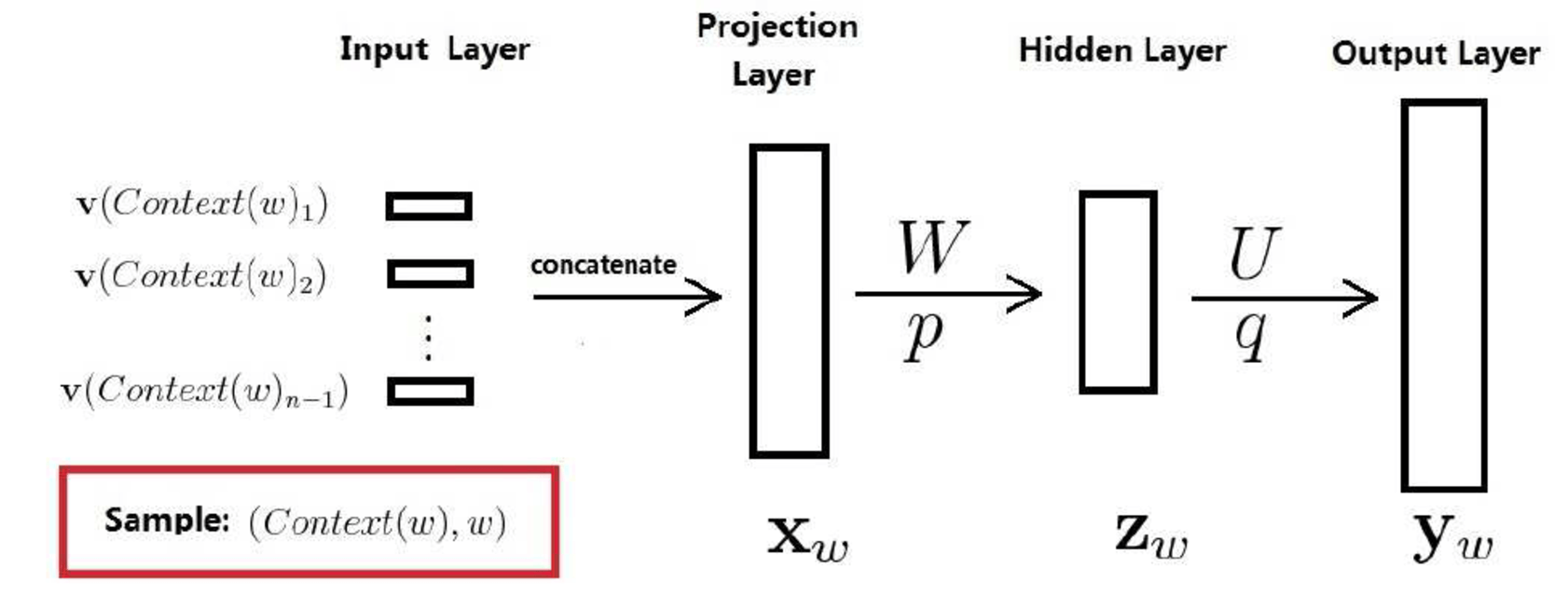• 训练样本为 (Context(w), w)，其中 $w \in Corpus$, $Context(w)$ 为其前面的 $n-1$ 个词
• $X_w$ 为直接将 $Context(w)$​ 收尾顺次相接得到的 $(n-1) \cdot m$ 长度的向量，其中 $m$ 为词向量长度
• $Z_w = \tanh (WX_w + p), \, y_w = Uz_w + q$.

• Word2vec 对网格结构的优化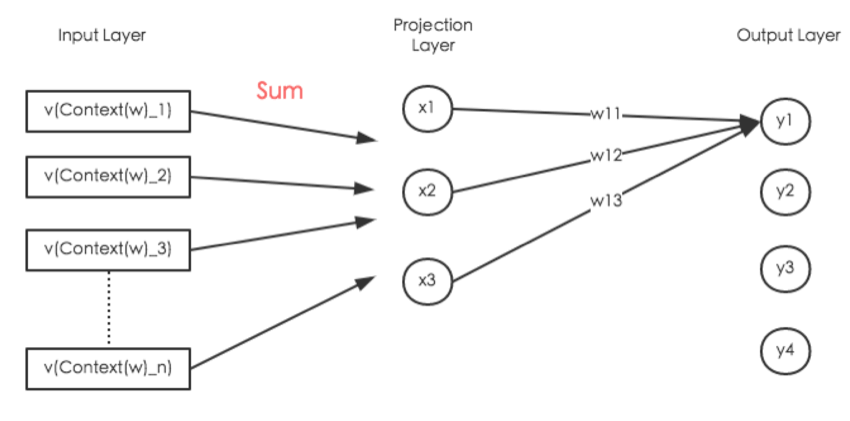• 对 Softmax 归一化的优化

• Hierarchical Softmax

• Negative Sampling

NCE posits that a good model should be able to differentiate data from noise by means of logistic regression.

Word2vec 采用的 Negative Sampling 是 NCE 的一种简化版本，目的是为了提高训练速度以及改善所得词的质量。相比于 Hierarchical Softmax，Negative Sampling 不再采用 Huffman 树，而是采用随机负采样

Word2vec 的作者们测试发现，最佳的分布是 $\frac 3 4$ 次幂的 Unigram Distribution，也就是说，选中语料库中词 $w$ 的概率为 $\dfrac {count(w)^{3/4}} {\sum_{u \in D}count(u)^{3/4}}$.

• Continous Bag-of-words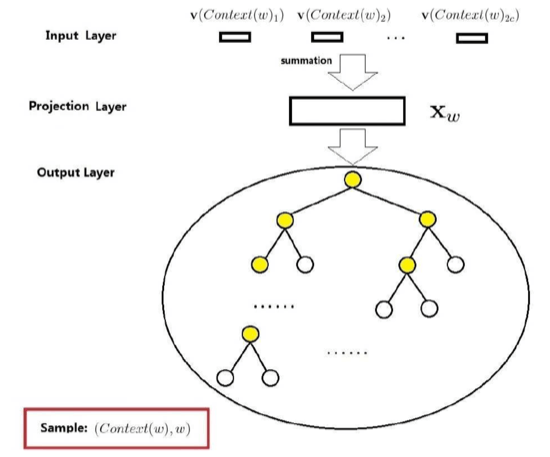• $Context(w)$: 由 $w$ 前后各 $c$ 个词构成
• 输入层：包含 $2c$ 个词的词向量
• 投影层：将 $2c$ 个词向量累加求和得到的 $X_w$
• 输出层：输出层对应的是一棵 Huffman 树。该 Huffman 树以语料中出现的词为叶子节点，以各词在语料中出现的次数当做权值构造出来的。该树中，叶子节点总共有 $N$ 个（$N = |D| := |Corpus|$）。

• $p^w$：从根节点到 $w$ 对应的那个叶子结点在树中的路径
• $l^w$：路径 $p^w$ 上包含的结点数目
• $p^w_1, p^w_2, \cdots, p^w_{l^w}$：路径 $p^w$ 上对应的结点
• $d^w_2, d^w_3, \cdots, d^w_{l^w}$：路径 $p^w$ 上的结点对应的 Huffman 编码
• $\theta^w_1, \theta^w_2, \cdots, \theta^w_{l^w}$：路径 $p^w$ 上非叶子结点对应的词向量

• 分为正类的概率：$\sigma(X_w^T\theta) = \dfrac 1 {1 + e^{-X_w^T\theta}}$
• 分为负类的概率：$1-\sigma(X_w^T\theta)$

• Skip-gram

Skip-gram 模型是已知当前词 $w$，对其上下文中的词 $Context(w)$ 进行预测。

### GloVe

• 在有些情况下，交叉熵损失函数有劣势。GloVe 模型采用了平方损失，并通过词向量拟合预先基于整个数据集计算得到的全局统计信息。
• 任意词的中心词向量和背景词向量在 GloVe 模型中是等价的。

### Encoder-Decoder

• 总览• Encoder (Usually RNN)

• Decoder

• 输出层的贪婪搜索不一定能得到最优输出序列

• 穷举搜索的开销过于庞大
• Beam Search!### Attention Mechanism

• 计算背景变量• 矢量化计算 (Query, Key & Value)

• 更新隐藏状态 (GRU)